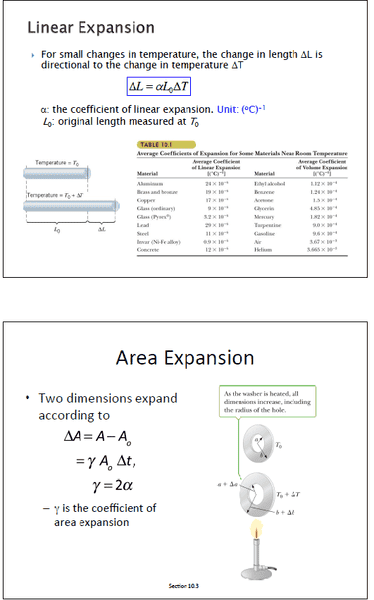# Thermal Expansion linear vs area expansion

• Searay330

#### Searay330

Member warned to use the formatting template for homework posts.this is a picture of my notes for thermal expansion for linear vs area.
my question is why does the area coefficient of expansion for the area = 2(liner coefficient of expansion).
any insight would be appreciated.

As an example to ponder, consider an object with a flat, square surface that is L units of length on each side. Apply the linear expansion concept to each dimension and work out an expression for the change in area. Then consider that the coefficient of linear expansion ##\alpha## is typically on the order of a few parts per million per degree C. Is there an obvious simplification?

im not sure the change in area would be equal to the new L2 and that is the only dimension that changes

im not sure the change in area would be equal to the new L2 and that is the only dimension that changes
No, the change in area would not equal L2.
If the original side of the square is L, the original area is L2.
If the new side length is ##L+\alpha L\Delta T##, what is new area? How much has the area increased by?

its increased by 2(αLΔT) one for each side

its increased by 2(αLΔT) one for each side